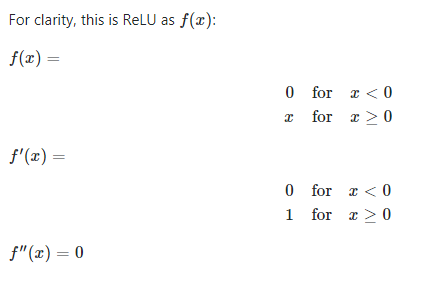# Backpropagation: In second-order methods, would ReLU derivative be 0? and what its effect on training?

##### By Pratik20, 4 months ago

ReLU is an activation function defined as h=max(0,a) where a=Wx+b

Normally, we train neural networks with first-order methods such as SGD, Adam, RMSprop, Adadelta, or Adagrad. Backpropagation in first-order methods requires the first-order derivative. Hence x is derived to 1.

But if we use second-order methods, would ReLU's derivative be 0? Because x is derived to 1 and is derived again to 0. Would it be an error? For example, with Newton's method, you'll be dividing by 0 (I don't really understand Hessian-free optimization, yet. IIRC, it's a matter of using an approximate Hessian instead of the real one).

What is the effect of this h″=0? Can we still train the neural network with ReLU with second-order methods? Or would it be non-trainable/error (nan/infinity)?Neural-network
Optimization
Backpropagation
Relu
##### Jennifer

Yes the ReLU second order derivative is 0. Technically, neither dy/dx nor d2y/dx2 are defined at x=0, but

we ignore that - in practice an exact x=0 is rare and not especially meaningful, so this is not a problem. Newton's method does not work on the ReLU transfer function because it has no stationary points. It also doesn't work meaningfully on most other common transfer functions though - they cannot be minimised or maximised for finite inputs.

When you combine multiple ReLU functions with layers of matrix multiplications in a structure such as a neural network, and wish to minimise an objective function, the picture is more complicated. This combination does have stationary points. Even a single ReLU neuron and a mean square error objective will have different enough behaviour such that the second-order derivative of the single weight will vary and is not guaranteed to be 0.

Nonlinearities when multiple layers combine is what creates a more interesting optimisation surface. This also means that it is harder to calculate useful second-order partial derivatives (or Hessian matrix), it is not just a matter of taking second order derivatives of the transfer functions.

The fact that  d2y/dx2 =0 for the transfer function will make some terms zero in the matrix (for the second order effect from same neuron activation), but the majority of terms in the Hessian are of the form ∂2E/∂xi∂xj where E is the objective and xi, xj are different parameters of the neural network. A fully-realised Hessian matrix will have N2 terms where N

N is number of parameters - with large neural networks having upwards of 1 million parameters, even with a simple calculation process and many terms being 0 (e.g. w.r.t. 2 weights in same layer) this may not be feasible to compute.

There are techniques to estimate effects of second-order derivatives used in some neural network optimisers. RMSProp can be viewed as roughly estimating second-order effects, for example. The "Hessian-free" optimisers more explicitly calculate the impact of this matrix.

#### How Artificial Intelligence Works and How To Make Career In AI?Oct 2nd (11:00 AM) 354 Registered
More webinars

#### Related Discussions

Running random forest algorithm with one variable

View MoreBOT
Agent(Online)
We're Online!

Chat now for any query